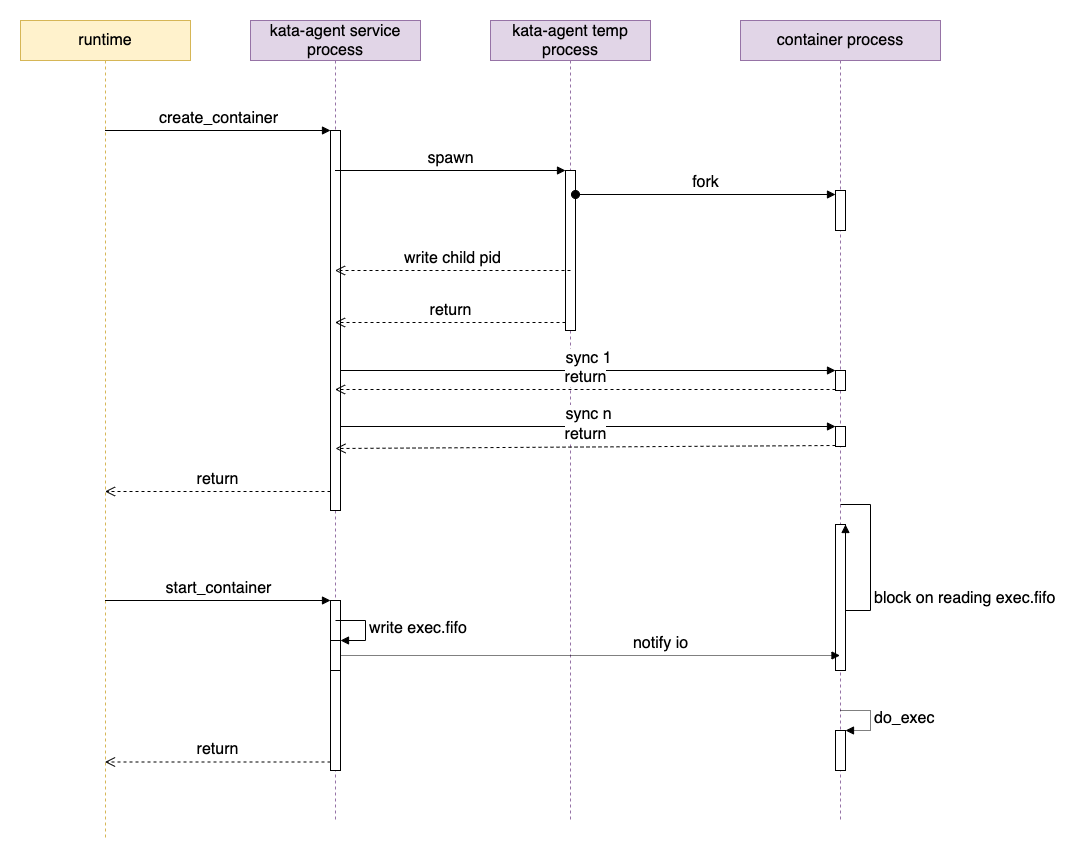# agent 代码解析

agent 可以作为 guest init 进程启动，也可以使用 systemd 等作为 init ，agent 作为普通进程启动。在这篇文章中，我们将以 agent 作为普通服务/进程启动，使用 systemd 作为 init 系统为例进行说明。

agent 项目又分为如下几个 crate：

• agent：代码位置 src/agent，即 agent 代码的根。
• rustjail： 类似 golang 中的 libcontainer 的角色。代码位置 src/agent/rustjail
• protocols：主要定义了 runtim <-> agent 之间的通信协议。代码位置 src/agent/protocols
• oci：主要是定义了 OCI 相关 spec 的数据结构。代码位置 src/agent/oci

## agent 进程

agent 的启动代码见 main.rs 的 main 函数即可。

agent 程序有两个作用：

• ttrpc服务：作为 agent 服务，通过 ttrpc 响应来自 shim 进程的请求
• 启动容器：启动容器时，agent 会通过运行 agent 二进制在新的进程中启动容器

### 启动 ttrpc 服务

main 函数会判断命令行参数，如果第一个参数是 init，表示是要启动一个新的容器；否则表示要启动 ttrpc 服务：

``````fn main() -> std::result::Result<(), Box<dyn std::error::Error>> {
let args: Vec<String> = env::args().collect();

// 如果是 kata-agent init，则准备进入创建容器的步骤
if args.len() == 2 && args == "init" {
rustjail::container::init_child();
exit(0);
}

.enable_all()
.build()?;

// 在 real_main 里启动 ttrpc 服务。
rt.block_on(real_main())
}
``````

TODO

### 启动容器流程

• create_container
• start_container

#### create_container/do_create_container

`do_create_container` 中主要有如下操作：

• rescan_pci_bus：查找新设备
• add_devices：更新设备列表，让 OCI spec 中的配置和 guest 中的设备同步
• update_container_namespaces：更新 OCI spec 中的 namespace
• update_device_cgroup：更新 device cgroup
• append_guest_hooks
• setup_bundle：创建、设置容器的 bundle 目录，创建 config.json 文件。

``````
let mut ctr: LinuxContainer =
LinuxContainer::new(cid.as_str(), CONTAINER_BASE, opts, &sl!())?;

let p = if oci.process.is_some() {
Process::new(
&sl!(),
&oci.process.as_ref().unwrap(),
cid.as_str(),
true,
pipe_size,
)?
} else {
return Err(anyhow!(nix::Error::from_errno(nix::errno::Errno::EINVAL)));
};

ctr.start(p).await?;
``````

#### ctr.start(p)

agent 创建容器进程的时候，会先启动 `kata-agent` 进程：

``````let exec_path = std::env::current_exe()?;
let mut child = std::process::Command::new(exec_path);
let mut child = child
.arg("init")
.stdin(child_stdin)
.stdout(child_stdout)
.stderr(child_stderr)
.env(INIT, format!("{}", p.init))
.env(NO_PIVOT, format!("{}", self.config.no_pivot_root))
.env(CRFD_FD, format!("{}", crfd))
.env(CWFD_FD, format!("{}", cwfd))
.env(CLOG_FD, format!("{}", cfd_log));

if p.init {
child = child.env(FIFO_FD, format!("{}", fifofd));
}

child.spawn()?;
``````

• `p.init`： Process 的 init 属性，如果是 true，表示启动的是容器的进程，如果是 false 表示是 exec RPC 启动的普通进程，这两种进程处理有区别。
• FIFO_FD 是容器 bundle 下的一个名为 `exec.fifo` 的文件，这个文件用于控制容器进程的启动。

``````let pid_buf = read_async(&mut pipe_r).await?;
let pid_str = std::str::from_utf8(&pid_buf).context("get pid string")?;
let pid = match pid_str.parse::<i32>() {
Ok(i) => i,
Err(e) => {
}
};
``````

``````let (prfd, cwfd) = unistd::pipe().context("failed to create pipe")?;
let (crfd, pwfd) = unistd::pipe().context("failed to create pipe")?;

let mut pipe_r = PipeStream::from_fd(prfd);
let mut pipe_w = PipeStream::from_fd(pwfd);
``````

`ctr.start()` 最后执行的一个比较重要的函数就是 `join_namespaces` 了，在这个函数中，会创建、启动真正容器进程。而真正的进程管理工作，则是在新启动的子 kata-agent 进程中实现的。也就是下面要介绍的 do_init_child 函数。

#### do_init_child

do_init_child 是 kata-agent 进程启动后，创建真正容器进程的处理函数。do_init_child 和 kata-agent 进程通过 cwfd/crfd 这对分别用于写入和读取的 fd 来通信（同步）。

``````// kata-containers/src/agent/rustjail/src/container.rs

fn do_init_child(cwfd: RawFd) -> Result<()> {
match fork() {
Ok(ForkResult::Parent { child, .. }) => {
let _ = write_sync(cwfd, SYNC_DATA, format!("{}", pid_t::from(child)).as_str());
// parent return
return Ok(());
}
Ok(ForkResult::Child) => (),
Err(e) => {
}
}

}
``````

`do_init_child` 函数最后部分代码如下：

``````
if init {
if init {
fifofd = std::env::var(FIFO_FD)?.parse::<i32>().unwrap();
}

... ...

let fd = fcntl::open(
format!("/proc/self/fd/{}", fifofd).as_str(),
OFlag::O_RDONLY | OFlag::O_CLOEXEC,
Mode::from_bits_truncate(0),
)?;
unistd::close(fifofd)?;
let mut buf: &mut [u8] = &mut ;
}

do_exec(&args);
``````

#### start_container/do_start_container

start_container 最终会执行到这个函数：

``````// kata-containers/src/agent/rustjail/src/container.rs
fn exec(&mut self) -> Result<()> {
let fifo = format!("{}/{}", &self.root, EXEC_FIFO_FILENAME);
let fd = fcntl::open(fifo.as_str(), OFlag::O_WRONLY, Mode::from_bits_truncate(0))?;
let data: &[u8] = &;
unistd::write(fd, &data)?;
info!(self.logger, "container started");
self.init_process_start_time = SystemTime::now()
.duration_since(SystemTime::UNIX_EPOCH)
.unwrap()
.as_secs();

self.status.transition(ContainerState::RUNNING);
unistd::close(fd)?;

Ok(())
}
``````

``````
fn do_exec(args: &[String]) -> ! {
let path = &args;
let p = CString::new(path.to_string()).unwrap();
let sa: Vec<CString> = args
.iter()
.map(|s| CString::new(s.to_string()).unwrap_or_default())
.collect();
let a: Vec<&CStr> = sa.iter().map(|s| s.as_c_str()).collect();

let _ = unistd::execvp(p.as_c_str(), a.as_slice()).map_err(|e| match e {
nix::Error::Sys(errno) => {
std::process::exit(errno as i32);
}
_ => std::process::exit(-2),
});

unreachable!()
}
``````

### 启动容器小结• create_container
• kata-agent service 运行 `kata-agent init` 启动容器临时进程
• 容器临时进程 fork()，父进程退出，将子进程的 pid 写回给 kata-agent service 进程
• 容器临时进程子进程准备容器运行环境，block 在读取 `exec.fifo`
• start_container
• kata-agent service 向容器的 `exec.fifo` 文件中写入数据
• 容器临时进程子进程继续执行，通过 execvp 启动容器的真实进程